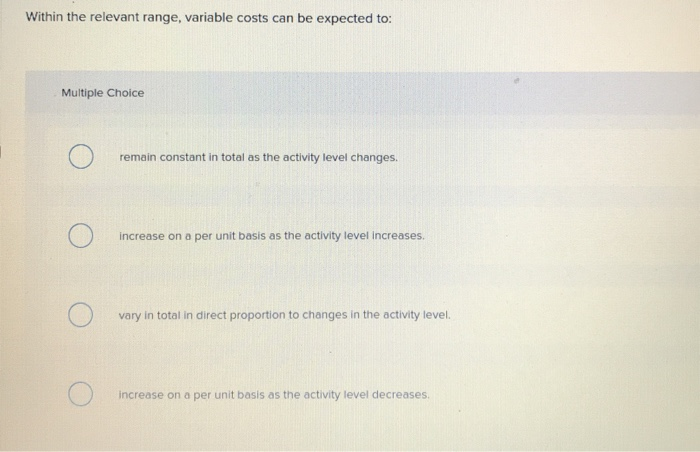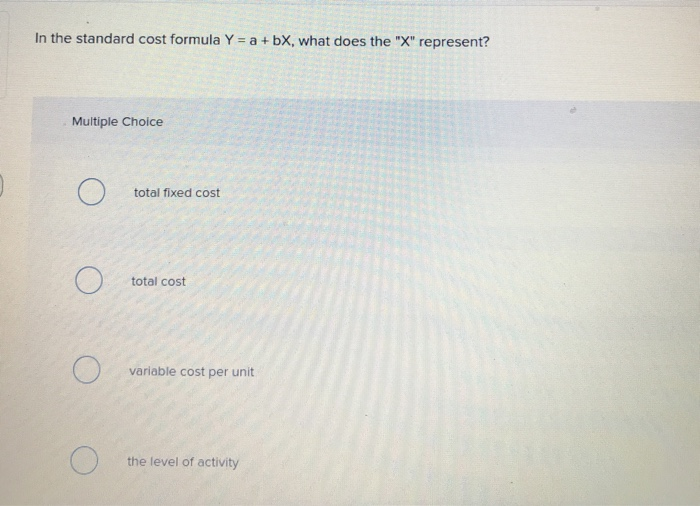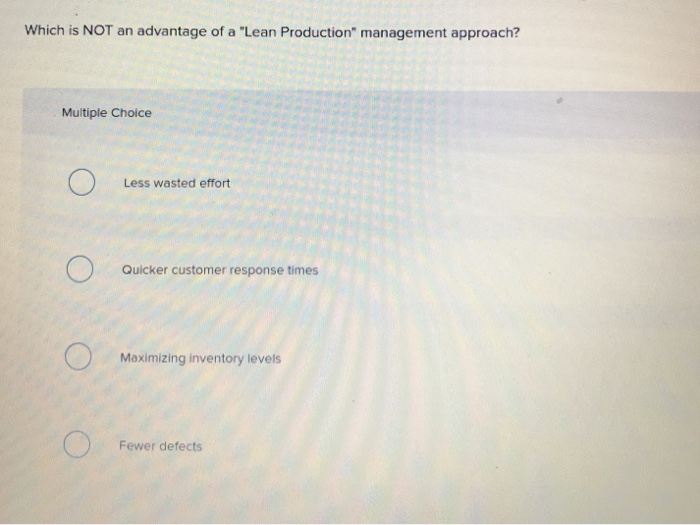# Within the relevant range variable costs can be expected to

1)
2)

3)1) 2) 3) Within the relevant range, variable costs can be expected to: Multiple Choice remain constant in total as the activity level changes. increase on a per unit basis as the activity level increases. vary in total in direct proportion to changes in the activity level. increase on a per unit basis as the activity level decreases. In the standard cost formula Y = a +bX, what does the “X” represent? Multiple Choice total fixed cost О O total cost variable cost per unit the level of activity Which is NOT an advantage of a “Lean Production” management approach? Multiple Choice O Less wasted effort Quicker customer response times Maximizing inventory levels Fewer defects

Question
1:

Explanation:
Within the relevant range, variable costs can be expected to
vary in total in direct proportion to changes in the activity
level. Fixed cost remains constant in total as the activity level
changes within the relevant range and also decreases on a per unit
basis. Variable cost remains constant on a per unit basis.
Question
2:

Explanation:
In the standard cost formula Y = a + bX,
Where Y = Total Cost
a = Fixed cost
b = variable cost per unit
X = the level of activity.
Question
3:

Explanation:
In lean management system, inventory are maintained at the lower
amount. This system focuses on quality, flexibility, time reduction
and quicker customer response times. Waste in lean management
system is defined as overproduction, unnecessary movement and high
levels of inventory. Therefore, maximizing inventory levels is not
an advantage of a lean production management approach.
All the best…

 Answer: vary in total in direct proportion to changes in the activity level.
 Answer: the level of activity.
 Maximizing inventory levels.